Technical Article

# Ratiometric Resistance Measurement Basics Using an Analog to Digital Converter

October 07, 2022 by Harry Trietley

## Learn the basics of ratiometric resistance measurements in analog to digital (A/D) converters, how it's measured, and example uses in a digital multimeter (DMM), microprocessor, and various resistive sensors.

A/D converters are ratiometric, that is, their result is proportional to the ratio of input voltage to reference voltage. This can be used to simplify resistance measurement.

The standard way to measure resistance is to pass a current through the resistor and measure its voltage drop (see Figure 1). Then, Ohm's Law (V = I x R) can be used to calculate the resistance from the voltage and current. The final output may be analog or digital.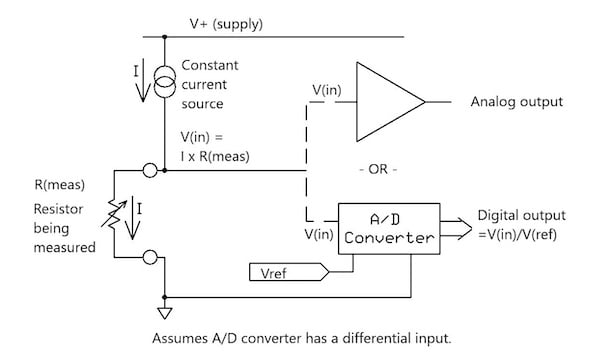##### Figure 1. An example block diagram showing resistance measurement.

The voltage is passed on to either an analog output circuit or an A/D converter. The current source circuit must be accurate, drift-free, and unaffected by the measured resistance and supply voltage variations. Designing such a circuit is not especially difficult but requires precise, stable components. The A/D converter, if used in this manner, needs an equally accurate and stable reference voltage.

### Ratiometric Resistance Measurement

If the same current is passed through two resistors, the ratio of their voltages will remain the same if the current changes. This can be expressed mathematically in Equation 1 as:

$$\frac{Voltage(2)}{Voltage(1)} = \frac{(I \times R2)}{(I \times R1)} = \frac{R2}{R1}$$

##### Equation 1.

We can use this information to develop an A/D converter system, as in Figure 2, that performs a ratiometric resistance measurement that does not need a constant current source or a precise reference voltage.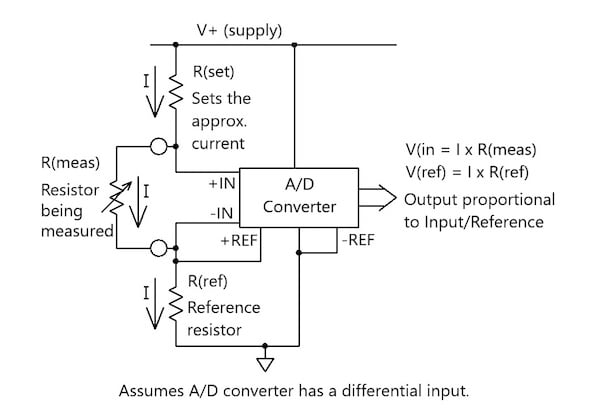##### Figure 2. Block diagram showing the use of an A/D converter for ratiometric resistance measurement.

Where:

• R(set) sets the approximate current (I), but the exact current varies as the measured resistance changes
• The measured voltage, V(in), equals I times the measured resistance, R(meas)
• The reference voltage, V(ref), equals I times the reference resistance, R(ref)

Overall, the digital result will be proportional to R(meas) / R(ref) regardless of the exact value of the current. As compared to the standard approach, no current source circuitry and no precision reference voltage are required. Only one component, R(ref), needs to be stable and precise.

It is important to note that this will work only if the A/D converter has a differential input, which shouldn't be a problem, seeing as most do. Most converters don’t have differential reference inputs, so R(ref) must connect to the circuit common. Both resistors must have the same current, thus, R(meas) is connected in series with R(ref). The configuration of Figure 2 is okay for a simple meter; however, it might not be suitable for sensor measurement systems with outputs connected to common. To solve that issue, you’d need an A/D converter with a differential reference input. We’ll cover that in the microprocessor section below.

With that in mind, let's take a look at the block diagram in Figure 3, which adds two new details.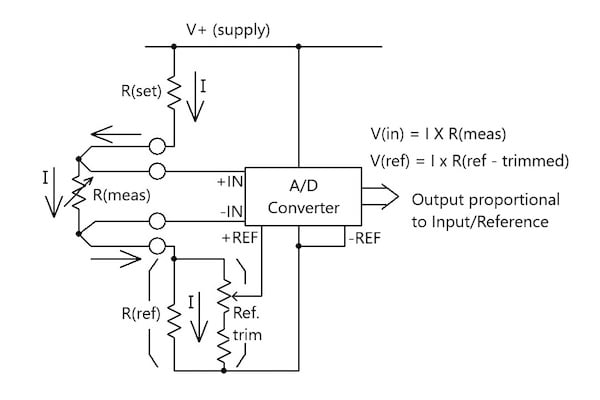##### Figure 3. Ratiometric measurement with details added: reference trim adjustment and optional four-wire resistance measurement.

The first addition is a reference trim adjustment. Without it, the conversion will only be as accurate as the reference resistor. For example, 0.05% accuracy would require a 0.05% or better resistor. With the trim, accuracy can be calibrated by measuring a high precision R(meas) and adjusting the trimmer for the proper digital output or reading. The fixed reference trim resistor should be higher than R(ref). The trimmer should be just a small percentage of the fixed resistor.

The second detail adds an optional four-wire (Kelvin) input measurement, sometimes needed for precise low-resistance measurements. Without it, the lead connection resistances add to R(meas), adding a fraction of an ohm. To see this, just take a standard multimeter, clip the ends of the test leads together, and measure the resistance. It will read a fraction of an ohm, not zero.

Furthermore, the four-wire connection supplies the current through one set of leads and uses a second pair to measure the input. No current flows through the measurement leads, so they drop no voltage. The measured voltage is truly I x R(meas), with no error due to lead resistances. High-precision meters usually include a four-wire resistance measurement capability.

### Resistance Measurement Example Using a Low-Cost DMM

With all of that information in hand, let's dive into an example using a low-cost DMM. Let's pretend I have a low-cost 3-1/2 digital multimeter bought for just a few dollars at a hardware store. I can’t fully explore its circuit because the IC chip is buried under epoxy; however, I ran a test, and it seems to operate this way using a non-constant current source. Table 1 below, has the results where the measured resistors had +1% tolerances:

##### Table 1.Data results with DMM set to the 200-ohm range.

R(meas) +1%

V(meas)

0 (short)

– – –

1.9 mA (approx.)

10.0 Ω

18.7 mV

1.87 mA

10.3 Ω

100 Ω

177.4 mV

1.74 mA

100.6 Ω

182 Ω

307.5 mV

1.68 mA

182.5 Ω

Table 2, on the other hand, show the data results when set to the 20 KΩ range.

##### Table 2. Data results with DMM set to the 20 KΩ range.

R(meas) +1%

V(meas)

I

0 (short)

– – –

22 uA (approx.)

0.00 KΩ

1.00 KΩ

22.4 mV

22.4 uA

1.00 KΩ

10.0 KΩ

133.5 mV

13.4 uA

9.99 KΩ

18.2 KΩ

178.2 mV

9.8 uA

182.7 KΩ

The results? The readings are all within the one percent tolerance even though the current varies.

As a note, my high-precision lab ohmmeter does not work this way. Its current remains precisely constant regardless of the measured resistance.

### Ratiometric Measurement Using a Microprocessor

Many microprocessors and microcontrollers include an A/D converter. Similar to Figure 3, Figure 4 shows an example block diagram of how an example microprocessor can be connected.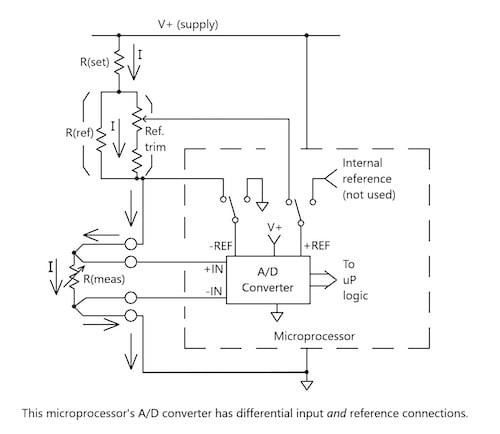##### Figure 4. Using an A/D converter with a differential reference input, you can connect the measured resistance to the circuit common.

Using an A/D converter with a differential reference input, you can connect the measured resistance to the circuit common. However, your microprocessor’s A/D may include a differential reference input. If so, you can take advantage of it to connect the measured resistor to the circuit common. As Figure 4 illustrates, the measured and reference resistors are interchanged.

Most microprocessors allow the A/D inputs to be switched using code. The plus reference can be switched to either internal or external reference, and the minus to external or common. If both are switched to external, the reference input becomes differential and does not need to be connected to common.

Furthermore, Figure 4 shows that R(meas) can now be connected to common and the reference resistor “floats.” The system can now connect both the input and the output to a common. Though the figure shows a four-wire input, for a two-wire input, just connect +IN to the current source and -IN to common.

### Ratiometric Measurement Basics for Resistive Sensors

Resistive sensors include thermistors, RTDs (resistive temperature detectors), and position-measurement potentiometers. Ratiometric measurement can be used for all, which we'll explain in the following sections.

#### Thermistors

Figure 5 shows some example thermistor package types.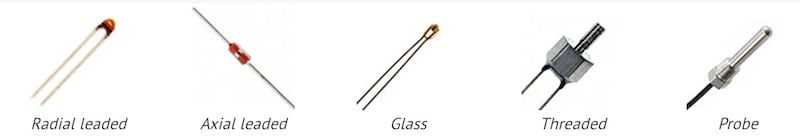##### Figure 5. Example thermistor package types. Image used courtesy of EE Power

The measurement part is simple—the thermistor becomes R(meas), and a two-wire input should work just fine. The difficult part is converting the resistance measurement to temperature. Both NTC (negative temperature coefficient) and PTC (positive temperature coefficient) thermistors are nonlinear and change resistance as the temperature changes.

Conversion requires either lookup tables or complex equations. Some analog techniques can approximately linearize the reading; however, only over narrow temperature ranges.

#### Resistive Temperature Detectors (RTDs)

RTDs are not low resistance, while many are 100 ohms at 0 °C, 200, 500, and 1,000-ohm versions also are common. A fraction of an ohm, though, can translate to an unacceptable temperature measurement error.

The sensitivity for platinum RTDS (the most common type) is about 0.4% per °C. In a 100-ohm device, 0.4 ohms of lead resistance becomes a 1 °C (1.8 °F) error, so a four-wire input is recommended. This may not be necessary at 500 or 1,000 ohms.

RTDs are not exactly linear with temperature, but their equations are fairly simple (which is beyond the scope of this article).

#### Potentiometers

Potentiometers are fairly simple. Basically, connect the (+) A/D input to the wiper and the (-) input to the low or counterclockwise end (-). The output will be proportional to the potentiometer’s position.

### Ratiometric Resistance Measurement Conclusion

The ratiometric resistance measurement concept is simple: put the same current through the measured and reference resistors, and the A/D output will be proportional to their ratio. We’ve expanded that with details that, we hope, will help you in your next designs.